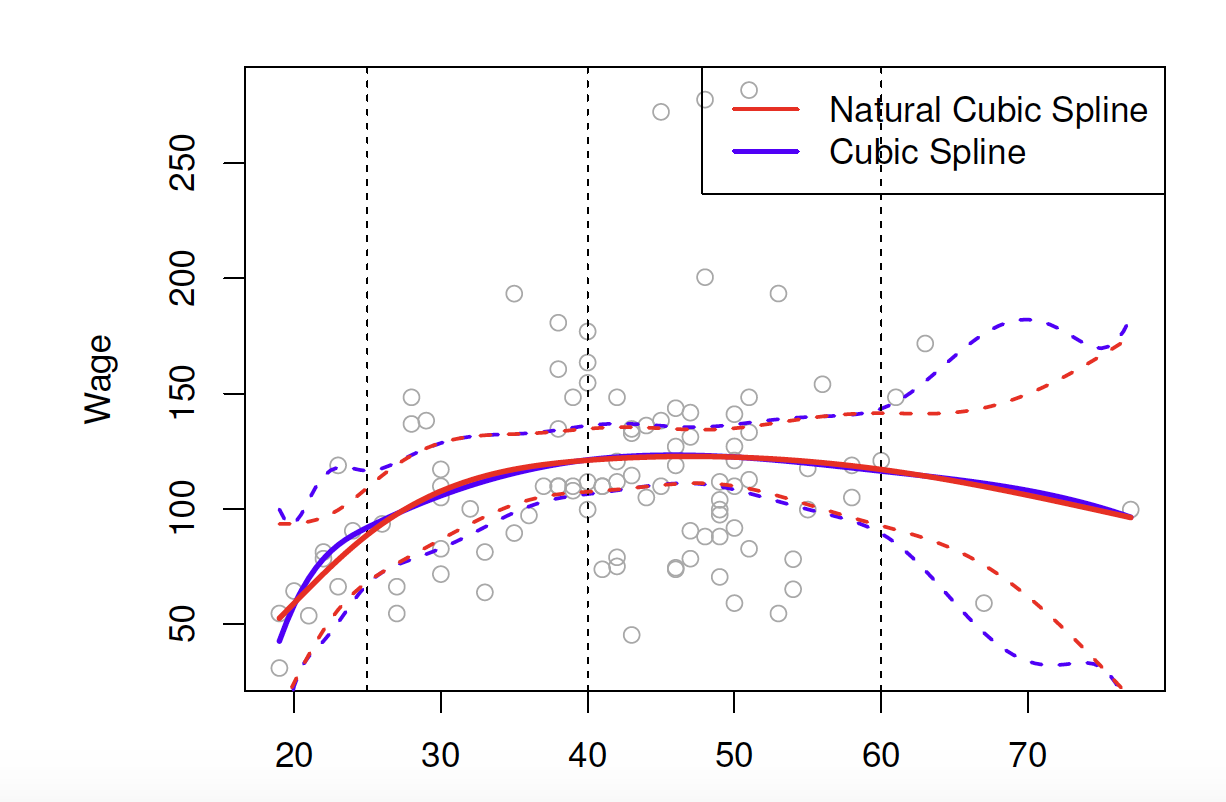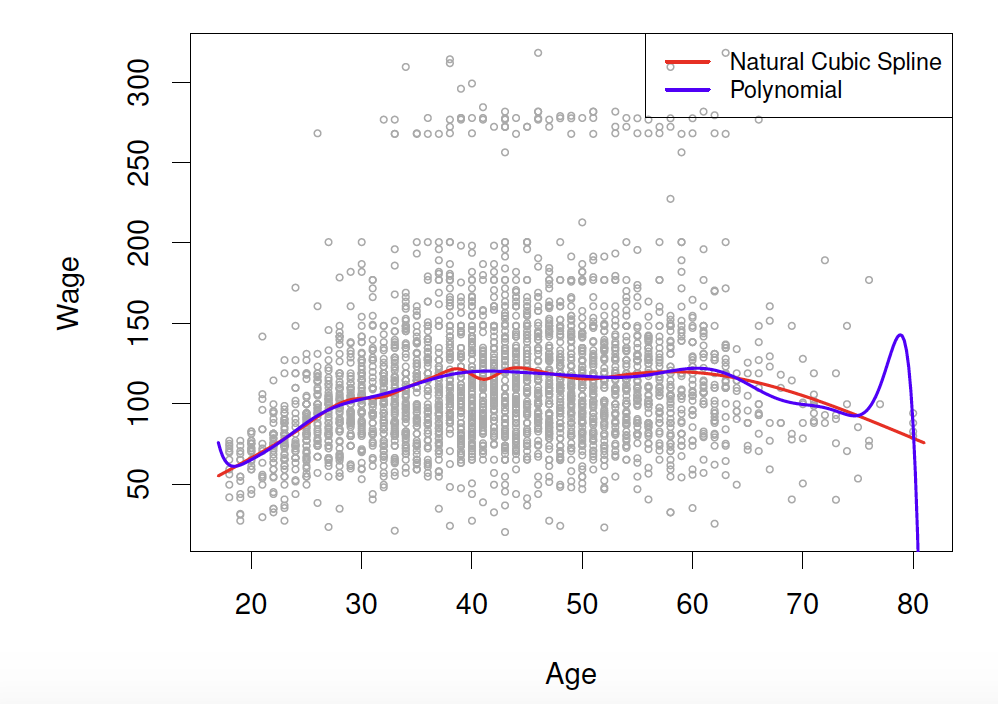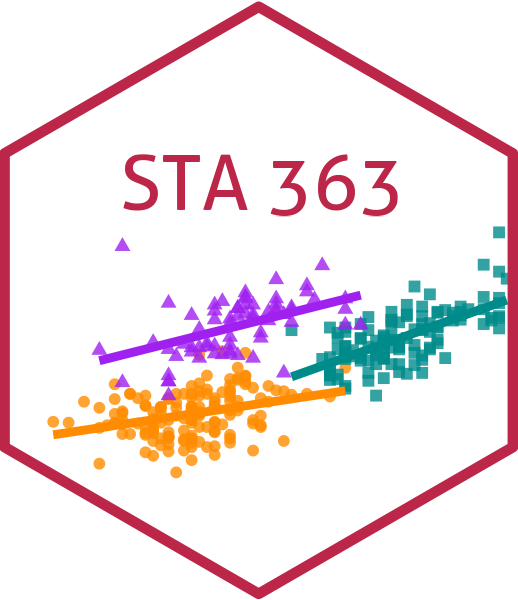# Non-linearity

Lucy D’Agostino McGowan

## Non-linear relationships

What have we used so far to deal with non-linear relationships?

• Hint: What did you use in Lab 02?
• Polynomials!

## Polynomials

$y_i = \beta_0 + \beta_1x_i + \beta_2x_i^2+\beta_3x_i^3 \dots + \beta_dx_i^d+\epsilon_i$

This is data from the Columbia World Fertility Survey (1975-76) to examine household compositions

## Polynomials

$y_i = \beta_0 + \beta_1x_i + \beta_2x_i^2+\beta_3x_i^3 \dots + \beta_dx_i^d+\epsilon_i$

• Fit here with a 4th degree polynomial

## How is it done?

• New variables are created ( $X_1 = X$, $X_2 = X^2$, $X_3 = X^3$, etc) and treated as multiple linear regression
• We are not interested in the individual coefficients, we are interested in how a specific $x$ value behaves
• $\hat{f}(x_0) = \hat\beta_0 + \hat\beta_1x_0 + \hat\beta_2x_0^2 + \hat\beta_3x_0^3 + \hat\beta_4x_0^4$
• or more often a change between two values, $a$ and $b$
• $\hat{f}(b) -\hat{f}(a) = \hat\beta_1b + \hat\beta_2b^2 + \hat\beta_3b^3 + \hat\beta_4b^4 - \hat\beta_1a - \hat\beta_2a^2 - \hat\beta_3a^3 -\hat\beta_4a^4$
• $\hat{f}(b) -\hat{f}(a) =\hat\beta_1(b-a) + \hat\beta_2(b^2-a^2)+\hat\beta_3(b^3-a^3)+\hat\beta_4(b^4-a^4)$

## Polynomial Regression

$\hat{f}(b) -\hat{f}(a) =\hat\beta_1(b-a) + \hat\beta_2(b^2-a^2)+\hat\beta_3(b^3-a^3)+\hat\beta_4(b^4-a^4)$

How do you pick $a$ and $b$?

• If given no other information, a sensible choice may be the 25th and 75th percentiles of $x$

## Application Exercise

$pop = \beta_0 + \beta_1age + \beta_2age^2 + \beta_3age^3 +\beta_4age^4+ \epsilon$

Using the information below, write out the equation to predicted change in population from a change in age from the 25th percentile (24.5) to a 75th percentile (73.5).

term estimate std.error statistic p.value
(Intercept) 1672.0854 64.5606 25.8995 0.0000
age -10.6429 9.2268 -1.1535 0.2516
I(age^2) -1.1427 0.3857 -2.9627 0.0039
I(age^3) 0.0216 0.0059 3.6498 0.0004
I(age^4) -0.0001 0.0000 -3.6540 0.0004
03:00

## Choosing $d$

$y_i = \beta_0 + \beta_1x_i + \beta_2x_i^2+\beta_3x_i^3 \dots + \beta_dx_i^d+\epsilon_i$

### Either:

• Pre-specify $d$ (before looking 👀 at your data!)
• Use cross-validation to pick $d$

Why?

## Polynomial Regression

Polynomials have notoriously bad tail behavior (so they can be bad for extrapolation)

What does this mean?

## Step functions

Another way to create a transformation is to cut the variable into distinct regions

$C_1(X) = I(X < 35), C_2(X) = I(35\leq X<65), C_3(X) = I(X \geq 65)$

## Step functions

• Create dummy variables for each group
• Include each of these variables in multiple regression
• The choice of cutpoints or knots can be problematic (and make a big difference!)

## Step functions

$C_1(X) = I(X < 35), C_2(X) = I(35\leq X<65), C_3(X) = I(X \geq 65)$

What is the predicted value when $age = 25$?

## Step functions

$C_1(X) = I(X < 15), C_2(X) = I(15\leq X<65), C_3(X) = I(X \geq 65)$

What is the predicted value when $age = 25$?

## Piecewise polynomials

Instead of a single polynomial in $X$ over it’s whole domain, we can use different polynomials in regions defined by knots

$y_i = \begin{cases}\beta_{01}+\beta_{11}x_i + \beta_{21}x^2_i+\beta_{31}x^3_i+\epsilon_i& \textrm{if } x_i < c\\ \beta_{02}+\beta_{12}x_i + \beta_{22}x_i^2 + \beta_{32}x_{i}^3+\epsilon_i&\textrm{if }x_i\geq c\end{cases}$

What could go wrong here?

• It would be nice to have constraints (like continuity!)
• Insert splines!

## Linear Splines

A linear spline with knots at $\xi_k$, $k = 1,\dots, K$ is a piecewise linear polynomial continuous at each knot

$y_i = \beta_0 + \beta_1b_1(x_i)+\beta_2b_2(x_i)+\dots+\beta_{K+1}b_{K+1}(x_i)+\epsilon_i$

• $b_k$ are basis functions
• \begin{align}b_1(x_i)&=x_i\\ b_{k+1}(x_i)&=(x_i-\xi_k)_+,k=1,\dots,K\end{align}
• Here $()_+$ means the positive part
• $(x_i-\xi_k)_+=\begin{cases}x_i-\xi_k & \textrm{if } x_i>\xi_k\\0&\textrm{otherwise}\end{cases}$

## Application Exercise

Let’s create data set to fit a linear spline with 2 knots: 35 and 65.

x
4
15
25
37
49
66
70
80
1. Using the data to the left create a new dataset with three variables: $b_1(x), b_2(x), b_3(x)$
2. Write out the equation you would be fitting to estimate the effect on some outcome $y$ using this linear spline
04:00

## Linear Spline

x
4
15
25
37
49
66
70
80

### ➡️

$b_1(x)$ $b_2(x)$ $b_3(x)$
4 0 0
15 0 0
25 0 0
37 2 0
49 14 0
66 31 1
70 35 5
80 45 15

## Application Exercise

Below is a linear regression model fit to include the 3 bases you just created with 2 knots: 35 and 65. Use the information here to draw the relationship between $x$ and $y$.

term estimate std.error statistic p.value
(Intercept) -0.3 0.2 -1.3 0.3
b1 2.0 0.0 231.3 0.0
b2 -2.0 0.0 -130.0 0.0
b3 -3.0 0.0 -116.5 0.0
07:00

## Linear Splines

$b_1(x)$ $b_2(x)$ $b_3(x)$
4 0 0
15 0 0
25 0 0
37 2 0
49 14 0
66 31 1
70 35 5
80 45 15

## Cubic Splines

A cubic splines with knots at $\xi_i, k = 1, \dots, K$ is a piecewise cubic polynomial with continuous derivatives up to order 2 at each knot.

Again we can represent this model with truncated power functions

$y_i = \beta_0 + \beta_1b_1(x_i)+\beta_2b_2(x_i)+\dots+\beta_{K+3}b_{K+3}(x_i) + \epsilon_i$

\begin{align}b_1(x_i)&=x_i\\b_2(x_i)&=x_i^2\\b_3(x_i)&=x_i^3\\b_{k+3}(x_i)&=(x_i-\xi_k)^3_+, k = 1,\dots,K\end{align}

where

$(x_i-\xi_k)^{3}_+=\begin{cases}(x_i-\xi_k)^3&\textrm{if }x_i>\xi_k\\0&\textrm{otherwise}\end{cases}$

## Application Exercise

Let’s create data set to fit a cubic spline with 2 knots: 35 and 65.

x
4
15
25
37
49
66
70
80
1. Using the data to the left create a new dataset with five variables: $b_1(x), b_2(x), b_3(x), b_4(x), b_5(x)$
2. Write out the equation you would be fitting to estimate the effect on some outcome y using this cubic spline
05:00

## Cubic Spline

x
4
15
25
37
49
66
70
80

### ➡️

b1 b2 b3 b4 b5
4 16 64 0 0
15 225 3375 0 0
25 625 15625 0 0
37 1369 50653 8 0
49 2401 117649 2744 0
66 4356 287496 29791 1
70 4900 343000 42875 125
80 6400 512000 91125 3375

## Cubic Spline

term estimate std.error statistic p.value
(Intercept) 1.172 8.282 0.141 0.900
b1 1.520 1.565 0.971 0.434
b2 0.040 0.075 0.528 0.650
b3 -0.001 0.001 -0.855 0.483
b4 0.001 0.002 0.635 0.590
b5 -0.006 0.007 -0.860 0.480

## Natural cubic splines

A natural cubic spline extrapolates linearly beyond the boundary knots

This adds 4 extra constraints and allows us to put more internal knots for the same degrees of freedom as a regular cubic spline## Natural Splines

ggplot(da, aes(x = x, y = value, color = name)) +
geom_point(alpha = 0.5) +
geom_vline(xintercept = c(4, 80), lty = 2) +
labs(x = "X",
y = expression(hat(y)),
color = "Spline")

## Knot placement

• One strategy is to decide $K$ (the number of knots) in advance and then place them at appropriate quantiles of the observed $X$
• A cubic spline with $K$ knots has $K+3$ parameters (or degrees of freedom!)
• A natural spline with $K$ knots has $K-1$ degrees of freedom

## Knot placement

Here is a comparison of a degree-14 polynomial and natural cubic spline (both have 15 degrees of freedom)